﻿ A New ZCS High Step-up DC-DC Converter

### A New ZCS High Step-up DC-DC ConverterOPEN ACCESSPEER-REVIEWED

## A New ZCS High Step-up DC-DC Converter

Majid Delshad1,, Nima rakian1

1Electrical Department, Khorasgan Branch, Islamic Azad University, Isfahan, Iran

### Abstract

In this paper, a new high step-up PWM soft single switched converter is introduced. The proposed converter does not need any auxiliary switch to provide soft switching condition which causes simplification of control circuit. In this converter, the switch is turned on ZCS condition and turned off at almost ZVS. One of the main advantages of this converter is the possibility of sharing the output power between the magnetic devices and operating at high power levels. The experimental results obtain from the proposed converter verify the theoretical analysis.

### At a glance: Figures

123
Prev Next

• Delshad, Majid, and Nima rakian. "A New ZCS High Step-up DC-DC Converter." International Transaction of Electrical and Computer Engineers System 1.1 (2013): 1-5.
• Delshad, M. , & rakian, N. (2013). A New ZCS High Step-up DC-DC Converter. International Transaction of Electrical and Computer Engineers System, 1(1), 1-5.
• Delshad, Majid, and Nima rakian. "A New ZCS High Step-up DC-DC Converter." International Transaction of Electrical and Computer Engineers System 1, no. 1 (2013): 1-5.

 Import into BibTeX Import into EndNote Import into RefMan Import into RefWorks

### 1. Introduction

High step-up DC-DC converters are vastly used in green energy systems such as photovoltaic systems, fuel cell systems and etc. Modern DC-DC converters operate in high switching frequency to reduce their size and weight, but by increasing switching frequency, switching loss increases. To solve this problem, soft switching techniques are required [1-6].

Quasi-resonant and resonant converters do not require any extra switch to provide soft switching conditions but these converters must be controlled by variable switching frequency which causes the complexity of control circuit and design of magnetic components [7, 8, 9].

Using active clamp circuit is another technique to provide soft switching condition and absorbing voltage spike across the switch. Control of these converters is also pulse width modulation (PWM). Disadvantages of this technique are switch current stress, duty cycle losses and using extra switch [10, 11, 12].

Furthermore, zero-voltage transition (ZVT) and zero-current transition (ZCT) are PWM controlled but for implementing of these converters, at least two switches are required [13, 14, 15].

Although all the declared advantages exist on soft single switched converters, they usually have a large number of passive components, which increases conductors losses [16, 17, 18].

In this paper a new boost PWM soft single switched converter without any auxiliary switch is introduced. In the proposed converter voltage and current stress does not increase significantly and additional components are not high. The switch is turned on under ZCS condition and turned off at almost ZVS. The proposed converter is PWM controlled therefore, the implementation of control circuit is simple. One of the advantages of this converter is the possibility of sharing the output power between the magnetic devices and operating at high power levels.

In this paper, the operation modes of the proposed converter are demonstrated in section II. In section III, the steady state analysis is presented. The Experimental result from a 100 watt prototype is presented in section IV to verify the theoretical analysis.

### 2. Circuit Configuration and Operation Principle of the Proposed Converter

2.1. Circuit Description

The proposed single switch converter is shown in Figure 1. Auxiliary circuit provides zero current switching (ZCS) condition at turn on and almost zero voltage switching (ZVS) at turn off for switch. S is the main switch and Cb is balancing capacitor. C1 and C2 are output capacitors and Cr is resonant capacitor. D1-D3 are output diodes. In this convertor n and m are turns ratio of flyback and forward transformers respectively. Also Lm1 and Lm2 are magnetizing inductance of flyback and forward transformers. Lr1 and Lr2 are coupled inductors in auxiliary circuit.

2.2. Operation Principle

In order to simplify the steady state analysis, the following assumptions are made.

All parasitic components are neglected.

The output capacitors are large enough, so these voltages are considered constant in a switching cycle.

The proposed circuit has five operating modes in one period. The key waveforms of the proposed converter are illustrated in Figure 2. The equivalent circuits for each operating interval are shown in Figure 3.

Before the first mode, it is assumed that the switch is off and therefore diodes D1 and D2 are on and D3 is off and energy transfers to the output through flyback transformer.

2.2.1. Mode1

This mode begins when the switch S turns on under ZCS condition due to series inductor Lr1. The following equations are established:(1)(2)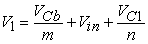(3)(4)

This mode ends when ISw reaches ILm2 . Duration of this mode can be calculated by following equation:(5)

2.2.2. Mode2

When D2 current reaches zero D3 conducts under ZCS condition and also, Lr1 start to resonate with Cr. The resonant capacitor voltage and resonant inductor current are as following: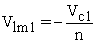(6)(7)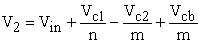(8)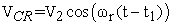(9)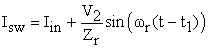(10)

Where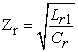(11)(12)

This mod ends when Vcr reaches zero. Duration of this mode is(13)

2.2.3. Mode3

When Vcr reaches zero this mode starts and D4 conducts under ZVS condition. Cr voltage is clamped at zero from this mode. Since the total ampere turns of Lr1 and Lr2 should stay constant.(14)

Furthermore, the Lr1 current is equal to the sum of the input current and Lr2 current, thus the following relations are obtained: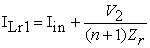(15)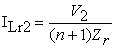(16)

This mode ends when Iin reaches to ILm1 and diode D1 turns off.

2.2.4. Mode4

In this mod switch is turned off and Cr starts to charge in resonant fashion. By turning the switch off, D1 is turned on and the ampere turn of Lr1 transfer to Lr2, and now, the Lr2 ampere turn is the sum of its previous ampere turn plus the Lr1 ampere turn as described by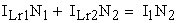(17)(18)(19)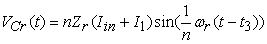(20)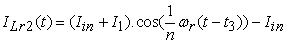(21)
Figure 3. Equivalent circuits for each operating interval of the proposed converter: (a):t0-t1, (b):t1-t2, (c):t2-t3, (d):t3-t4, (e):t4-t0, (The gray colored elements do not conduct current)

This mode ends when Vcr charges. Cr voltage can be calculated as following: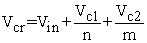(22)

2.2.5. Mode5

When Lr2 current reaches zero diode D4 is turned off under ZCS condition. In this mode Vcr remains constant and similar to previous mode, magnetizing inductances discharge to the output.

### 3. Analysis of Proposed Converter

3.1. Voltage dc Gain

In this section, by writing volt-sec balance for flyback and forward transformers and writing KVL in output loop the following relations are obtained.(23)(24)(25)

Thus VC1, VC2, VCb are obtained from equations (26) to (28).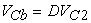(26)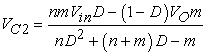(27)(28)

Finally, the gain of proposed converter is calculated below: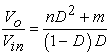(29)

In Figure 4 the proposed converter gain compared with conventional boost converter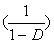. As seen in Figure 4, gain of proposed converter is higher than conventional isolated and non isolated boost converter.

3D plot of gain versus turn ratios and duty cycle is shown in Figure 5.

3.2. Soft Switching Condition

In this converter, Lr1 provides ZCS condition for the switch turn on instant. This inductor can be chosen according to , as follows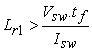(30)

Also, ZVS condition for the switch turn off instant is provided by Cr. Thus the value of this capacitor can be selected like snubber capacitors , according to the following relation: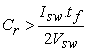(31)

Therefore, Lr1 and Cr can be obtained from Figure 6 and Figure 7 versus various output power.

3.3. Stress of Switching Devices

The maximum voltage stress across the main switch and diodes can be obtained from (32) to (36).(32)(33)(34)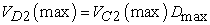(35)(36)

### 4. Experimental Results

The experimental results are presented in this section to verify the effectiveness of the proposed converter. The proposed converter specifications are listed in Table 1. Figure 8 and Figure 9 show the waveform of drain-source voltage, gate-source voltage and current of main switch to illustrate the ZCS feature. It can be observed that the main switch current is zero at the turn on instant. Also can be seen almost ZVS at turn off instant.

#### Table 1.Component used in the prototypeDownload asPowerPoint Slide

Veiw figureView current table in a new window

Figure 8. Drain-source voltage and current (dashed) waveforms of main switch S (vertical scale 25V/div or 2.5A/div, time scale 1µs/div)
Figure 9. Drain-source voltage and current (dashed) waveforms of main switch S (vertical scale 25V/div or 2.5A/div, time scale 1µs/div)

### 5. Conclusion

In this paper, a new single soft switched isolated PWM converter is proposed. The flyback transformer which is connected to the output in series leads to high voltage gain and less voltage stress on the power devices. The auxiliary circuit contains two coupled inductors and one snubber capacitor. In this converter, switch is turned on under zero current switching and turn off under almost zero voltage switching. One of the main advantages of this converter is the possibility of sharing the output power between the magnetic devices and operating at high power levels. Switch is PWM controlled which simplifies the control implementation. The simulation results obtained from the proposed converter verifies the theoretical analysis.

### References

  Bor-Ren Lin, Ming-Hung Yu, Jyun-Ji Chen “Interleaved Series-Parallel Flyback Converter with High Power Factor”. International Review of Electrical Engineering (IREE), Vol. 5, n. 4, Agugust 2010, pp. 1390-1397.In article  Bor-Ren Lin, Po-Li Chen, Jyun-Ji Chen“Analysis and Implementation of an Interleaved Isolated DC-DC Converter”. International Review of Electrical Engineering (IREE), Vol. 5. n.3, june2010, pp. 849-857.In article  M. Delshad“A New Single Switch Buck-Boost PWM DC-DC Converter”. International Review of Electrical Engineering (IREE), Vol. 4. n. 5, 2010, pp. 725-729.In article  M. Delshad “A Soft Switching Six Switch Current-Fed Converter with Voltage Doubler” International Review of Electrical Engineering (IREE), Vol. 4. n. 3, 2010, pp. 374-379.In article  M. Delshad, H. Farzanehfard “A New Soft Switching Isolated Buck-Boost PWM Converter”. International Review of Electrical Engineering (IREE), Vol. 3. n. 5, 2009, pp. 829-836.In article  J. Dudrik, ZVZCS PS-PWM Full Load Range DC-DC Converter, International Review of Electrical Engineering (IREE), vol. 1 n. 3, February 2007.In article  F. W. Combrink, H. T. Mouton, J. H. R. Enslin, and H. Akagi, “Design optimization of an active resonant snubber for high power IGBT converters,” IEEE Trans. Power Electron., vol. 21, no. 1, pp. 114-123, Jan. 2006.In article CrossRef  Y. Gu, Z. Lu, Z. Qian, X. Gu, and L. Hang, “A novel ZVS resonant reset dual switch forward DC–DC converter,” IEEE Trans. Power Electron., vol. 22, no. 1, pp. 96-103, Jan. 2007.In article CrossRef  Y. S. Lee and G. T. Cheng, “Quasi-resonant zero-current-switching bidirectional converter for battery equalization applications,” IEEE Trans. Power Electron, vol. 21, no. 5,pp. 1213-1224, Sep. 2006.In article CrossRef  S.K Han, H.K Yoon, G.W Moon; M.J Youn, Y.H Kim; K.H Lee, A new active clamping zero-voltage switching PWM current-fed half-bridge converter, IEEE Transactions on Power Electronics, Vol 20, November. 2005, pp.1271-1279.In article CrossRef  Y.K Lo, T.S Kao, J.Y Lin; Analysis and Design of an Interleaved Active-Clamping Forward Converter, IEEE Transactions on Industrial Electronics, Vol 54, August 2007, pp. 2323-2332.In article  T.F Wu, Y.S Lai, J.C Hung, Y.M. Chen, “Boost Converter With Coupled Inductors and Buck–Boost Type of Active Clamp” Vol 55, January. 2008, pp.154-162.In article  Adib, E.; Farzanehfard, H.; “Family of isolated zero-voltage transition PWM converters”vol.1,no. 1, 2008, pp. 144-153.In article  Adib, E.; Farzanehfard, H.; “Family of zero current zero voltage transition PWM converters” vol.1, no.2, 2008, pp. 214-223.In article  Adib, E.; Farzanehfard, H.; “Zero-Voltage-Transition PWM Converters With Synchronous Rectifier” vol. 25, no.2, 2010, pp. 214-223.In article  L. R. Barbosa, J. B. Vieira, L. C. Freitas, M. S. Vilela, and V. J. Farias,“A buck quadratic PWM soft-switching converter using a single activeswitch,” IEEE Trans. Power Electron., vol. 14, no. 3, May 1999, pp. 445-453.In article CrossRef  T. F. Wu and S. A. Liang, “A systematic approach to developing singlestagesoft switching PWM converters,” IEEE Trans. Power Electron.vol. 16, no. 5, Sep. 2001, pp. 581-593.In article CrossRef  E. S. da Silva, L. R. Barbosa, J. B. Vieira, L. C. Freitas, and V. J. Farias, “An improved boost PWM soft-single-switched converter with low voltage and current stresses,” IEEE Trans. Ind. Electron., vol. 48,no. 6, Dec. 2001, pp. 1174-1179.In article CrossRef  N. Mohan, T. M. Undeland, andW. P. Robbins, “Power Electronics”, 3rd ed. New York: Wiley, 2003, pp. 682-690.In article